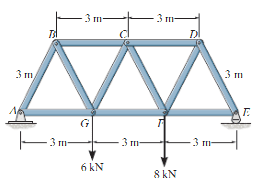# Statics: Tension or Compression?

• Elfrid Payton

#### Elfrid Payton

When examining a member, how do I tell whether it is undergoing tension or compression?

It's more of a general question, but since a visual might help with the explanation, this could be useful:Elfrid Payton said:
When examining a member, how do I tell whether it is undergoing tension or compression?
You can calculate it.
Sometimes intuition and some rough analysis are sufficient, sometimes they are not.

mfb said:
You can calculate it.
Sometimes intuition and some rough analysis are sufficient, sometimes they are not.

The calculations are not the problem. Figuring out whether the members are undergoing tension or compression for the FBDs is what I'm having trouble with.

The calculations will give you negative or positive values. There is no need to know that in advance.

## 1. What is statics?

Statics is a branch of mechanics that deals with the study of objects at rest or in a state of constant motion.

## 2. What is tension in statics?

Tension is a type of force that acts to stretch or elongate an object. In statics, tension occurs when a force is applied in opposite directions on an object, causing it to be pulled apart.

## 3. What is compression in statics?

Compression is a type of force that acts to push or compress an object. In statics, compression occurs when a force is applied in opposite directions towards each other, causing the object to be squeezed.

## 4. How do you determine if a force is causing tension or compression?

To determine if a force is causing tension or compression, you must look at the direction in which the force is applied. If the force is pulling or stretching an object, it is causing tension. If the force is pushing or compressing an object, it is causing compression.

## 5. What are some real-world examples of tension and compression in statics?

Examples of tension in statics include the tension in a rope or cable used to support a bridge or the tension in the strings of a guitar. Examples of compression in statics include the compression in the pillars of a building or the compression in the legs of a chair when someone sits on it.